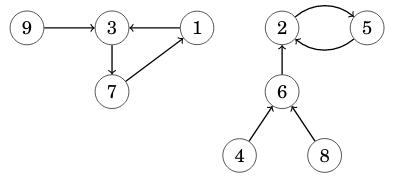CSES - Functional Graph Distribution
• Time limit: 1.00 s
• Memory limit: 512 MB
A functional graph is a directed graph where each node has outdegree $1$. For example, here is a functional graph that has $9$ nodes and $2$ components:Given $n$, your task is to calculate for each $k=1 \dots n$ the number of functional graphs that have $n$ nodes and $k$ components.

Input

The only input line has an integer $n$: the number of nodes.

Output

Print $n$ lines: for each $k=1 \dots n$ the number of graphs modulo $10^9+7$.

Constraints
• $1 \le n \le 5000$
Example

Input:
3

Output:
17
9
1